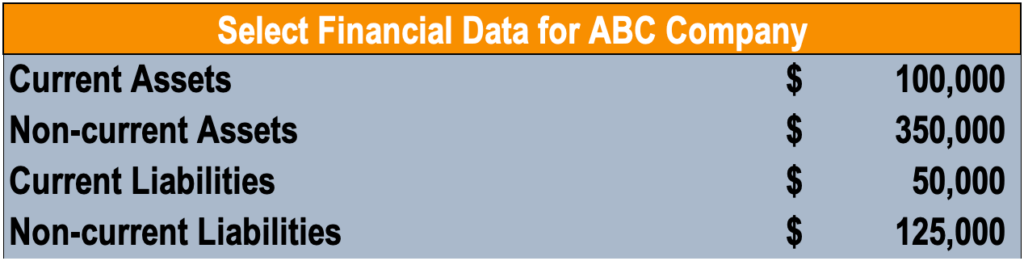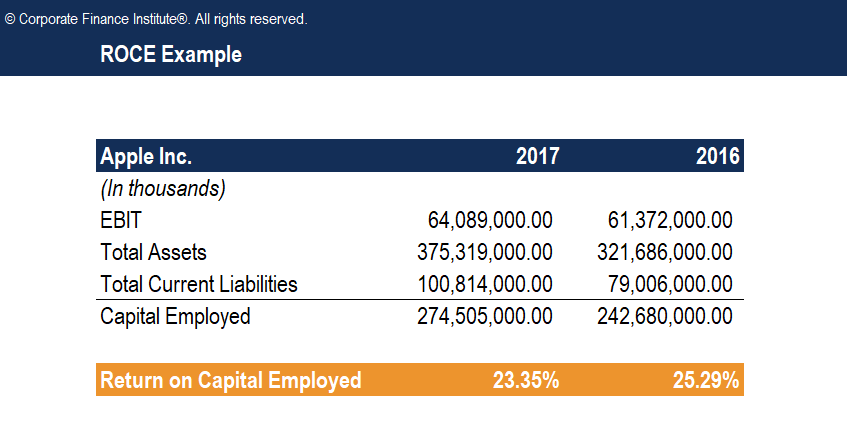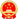# ROCE and Capital Employed

Hits: 4

## What is Capital Employed?

Capital employed refers to the amount of capital investment a business uses to operate and provides an indication of how a company is investing its money. Although capital employed can be defined in different contexts, it generally refers to the capital utilized by the company to generate profits. The figure is commonly used in the Return on Capital Employed (ROCE) ratio to measure a company’s profitability and efficiency of capital use.

### Formula

This metric can be calculated in two ways:

##### Capital Employed = Total Assets – Current Liabilities

Where:

• Total Assets are the total book value of all assets.
• Current Liabilities are liabilities due within a year.

or

##### Capital Employed = Fixed Assets + Working Capital

Where:

• Fixed Assets, also known as capital assets, are assets that are purchased for long-term use and are vital to the operations of the company. Examples are property, plant, and equipment (PP&E).
• Working Capital is the capital available for daily operations and is calculated as current assets minus current liabilities.

Note: The formula chosen should be consistently applied (do not switch between formulas when conducting trend analysis or peer comparisons) as the calculation differs depending on which formula is used. Generally, total assets minus current liabilities is the most commonly used formula.

Capital employed is very similar to invested capital, which is used in the ROIC calculation. Capital employed is found by subtracting current liabilities from total assets, which ultimately gives you shareholders’ equity plus long-term debts. Instead of using capital employed at an arbitrary point in time, some analysts and investors may choose to calculate ROCE based on the average capital employed, which takes the average of opening and closing capital employed for the time period under analysis.

### Sample Calculation

Mary is looking to calculate the capital employed of ABC Company, compiling the following information:Using the first formula above, Mary calculates the amount as follows:

### Interpreting Capital Usage

This metric provides an insight into how well a company is investing its money to generate profits. Although the figure varies depending on the formula used, the underlying idea remains the same.

The number in itself is seldom used by analysts. It is commonly used in conjunction with earnings before interest and tax (EBIT) in the return on capital employed (ROCE) ratio. As will be explained below, ROCE is a commonly used ratio by analysts for assessing the profitability of a company for the amount of capital used.

### Return on Capital Employed

Return on capital employed (ROCE) is a profitability ratio that measures the profitability of a company and the efficiency with which a company is using its capital. The ROCE is considered one of the best profitability ratios, as it shows the operating income generated per dollar of invested capital. The formula for ROCE is as follows:

### Example of ROCE

Recall that the capital employed for ABC Company in our example above is \$400,000. Assuming that earnings before interest and taxes figure of ABC Company is \$30,000, what is the ROCE?

##### ROCE = \$30,000 / \$400,000 = 0.075 = 7.5%

For every dollar of invested capital, ABC Company generated 7.5 cents in operating income.

Here is a screenshot of the template:粤ICP备2022015479号-1 All Rights Reserved © 2017-2023